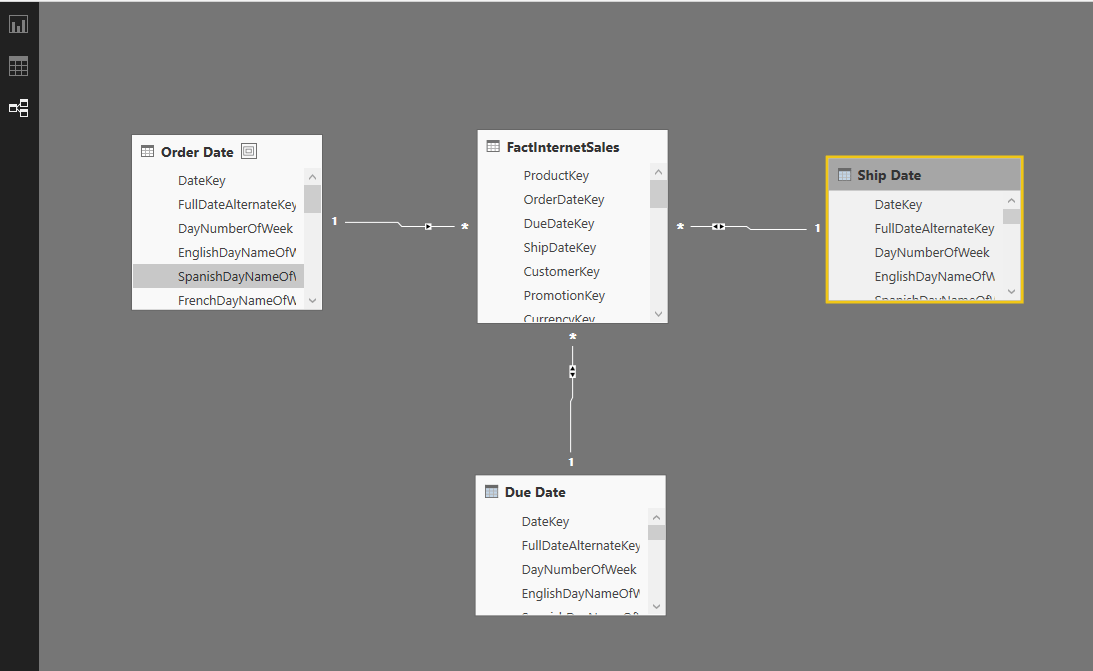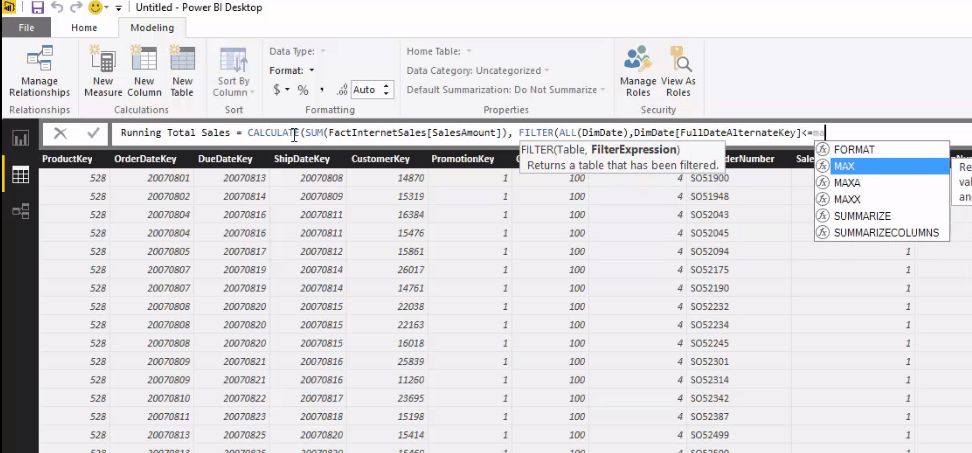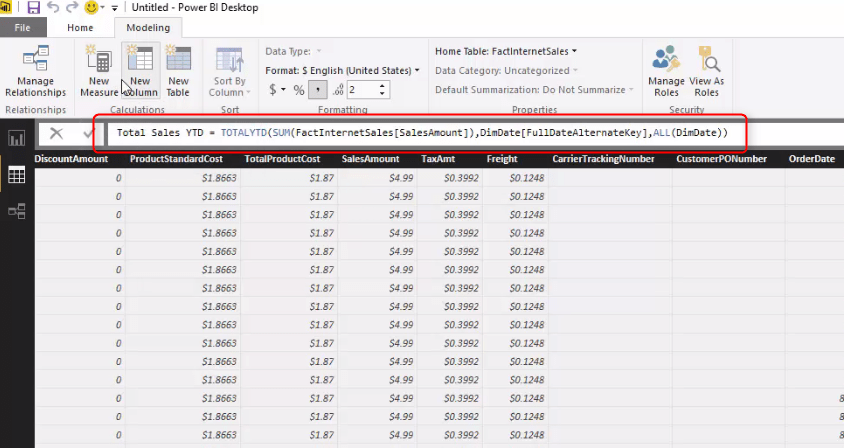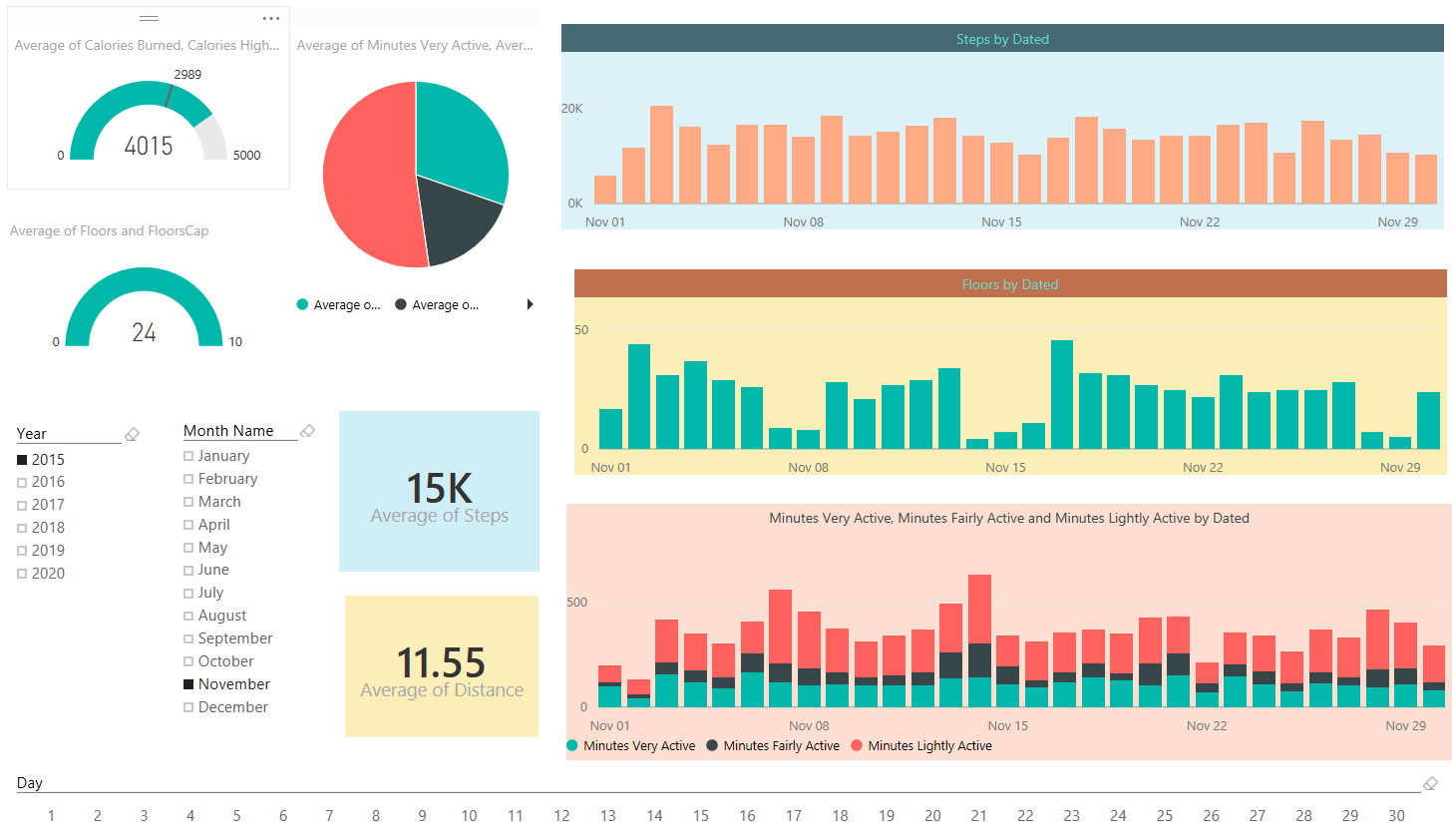# Lessons Archive

## Scenarios of Using Calculated Tables

Length: 9 minutesCalculated tables first introduced in September 2015 update of Power BI Desktop. The name speak for itself; these are tables created by calculation. As these are in-memory tables their calculation is based on DAX (Data Analysis eXpression language). There are many benefits of using Calculated tables, such as using them for role playing dimensions (for example having more than one date dimension in a model), There are some DAX functions and expressions that returns a table as a result, and using them as table in your model sometimes is really helpful. for example you might want to create a table for top 10 customers and then use that as the main source table in many reports. In this lesson I’ll explain to you some use cases of calculated tables.

## Modeling Best Practices

Length: 5 minutesThis quick lesson covers best practices and tips that you have to follow when designing a data model in Power BI. Tips includes naming standards, data types considerations, formatting and categories. relationship, filtering data rows before using it, creating star schema model, and some other tips that helps performance and readability of the data model for end user.

## Rolling 12 Months Calculation

Length: 14 minutesIn this lesson you will learn how to write more complex DAX expressions through an example of calculating average rolling 12 months sales. for this example we will be using Calculate and some other functions to calculate sum or rolling 12 month, and number of months, and then dividing them. You will learn functions such as; CountRows, Divide, LastDate, NextDay, DatesBetween, and Calculate in this lesson.

## CALCULATE function for Time Intelligence

Length: 6 minutesIn addition to existing DAX functions for time such as TotalYTD and QTD, there are some expression that needs another function. In this lesson you will learn about CALCULATE function. You will learn how this function can be used with combination of a filter and expression to achieve a time intelligence measure. You will learn this through examples of creating running total and same period last year measures.

## Secret of Time Intelligence Functions in Power BI

Length: 13 minutesTime Intelligence functions in DAX are helpful in time analysis and creating calculations for year to date, quarter to date for calendar or fiscal categories. In this lesson you will learn how to use functions such as TotalYTD and TotalQTD combined with a date dimension to create measures in DAX and Power BI. You will also learn the difference between Power Pivot which allows you to set a date table, and Power BI which you have to add the ALL(<dimDate>) filter in all time intelligence measures.

## Calculated Columns and Measures

Length: 30 minutesLearn how to create Calculated Columns and Measures in DAX. You will learn differences between Row Context and Filter Context. and you will learn in which conditions you have to use Measures and where you have to use calculated columns. You will learn some DAX basics in this video as well. You will learn functions such as Related and RelatedTable in this lesson as relationship functions in DAX.

## Fitbit Data Integration with Power Query

Length: 16 minutesThis is real use case for Power Query through an example. In this example you will learn how custom functions can be used with parameters to expand a data transformation applied on a CSV file to all files under a folder. The solution implemented with this method can be refreshed and pick the new data set anytime a new file appears in the source folder. You will learn how to apply simple data transformation in Power Query, how to change the whole transformation set to a function utilizing a parameter, and how to search through files in a folder, and apply function on all files and combine the whole result set.